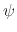Next: Implementation Details Up: Constant-pH Simulations 1 Previous: Constant-pH Simulations 1

## Overview and Theoretical Background

Constant-pH MD is a simulation methodology specially formulated for the treatment of variable protonation states. This is to be contrasted with conventional force-field based MD simulations, which generally treat protonation states by assuming they are fixed. Consider, for example, a protein with two titratable residues which may both be either protonated or deprotonated (Figure 13); the system has four possible protonation states. In the conventional route, the user must enumerate these possibilities, construct distinct topologies, and then simulate the cases individually. The simulations for each state must then be connected by either asserting knowledge about the system (e.g., by assuming that only certain states are of biological importance) or by performing additional simulations to probe transitions between states directly (e.g., by performing free energy calculations). In a constant-pH MD simulation, knowledge of the transformations is not assumed and is instead actively explored by interconverting between the various protonation states. This is especially useful when the number of protonation states is extremely large and/or prior information on the importance of particular states is not available.In formal terms, conventional MD samples from a canonical ensemble, whereas constant-pH MD samples from a semi-grand canonical ensemble. The new partition function,pH(91)

is essentially a weighted summation of canonical partition functions,, each of which are defined by an occupancy vector,. The elements ofare either one or zero depending on whether a given protonation site is or is not occupied, respectively. For a vector of length, the set of all protonation states,, has at mostmembers. In order to sample from the corresponding semi-grand canonical distribution function, a simulation must explore both the phase space defined by the canonical paritition functions and the state space defined by the different occupancy vectors. The fraction of simulation time spent in each state is dictated by the weights in the summation and these depend on the pH and the number of protons,, in the system (i.e., the sum of the elements in).

Although a constant-pH MD system may contain any number of titratable protons, the base transformation is always the movement of one proton from a molecule into a bath of non-interacting protons in solution.'' For a generic chemical species A, this corresponds to the usual deprotonation reaction definition, except with fixed pH:In the language of statistical mechanics the species HA and Arefer to all terms in Eq. (92) which do and do not, respectively, contain the specific proton in question (i.e., the particular element ofis one or zero). By taking out a factor of, this can be re-written aspHand then recast as a statistical mechanical analog of the Henderson-Hasselbalch equation by recognizing thatis just the ratio of deprotonated / protonated fractions of species A. The protonated fraction is then(92)

In practice,can be calculated from a simulation by simply counting the fraction of time spent in state HA (e.g., the fraction of time a specific element ofis one). Note also that pis formally a pH dependent function unless the system only contains one proton (or type of proton).

In most experimental contexts, a different form of Eq. (93) is used which is often referred to as a generalized'' Hill equation. This corresponds to a specific choice of pH dependence such that

 pThe constantis then known as the Hill coefficient and the so-called apparent p, p, generally corresponds to the inflection point of a plot of. Both quantities are usually determined by non-linear regression afterhas been determined at different pH values.Next: Implementation Details Up: Constant-pH Simulations 1 Previous: Constant-pH Simulations 1
http://www.ks.uiuc.edu/Research/namd/